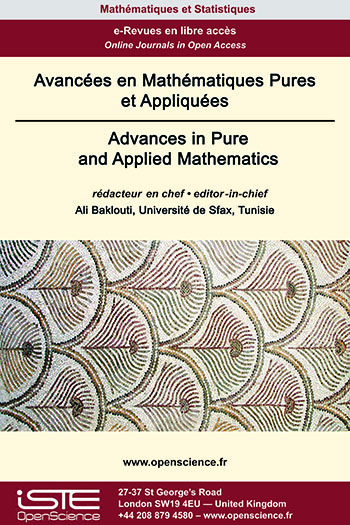# Vol 13 - Issue 4 (September 2022)

## List of Articles

Existence and multiplicity of solutions to a nonlocal elliptic PDE with variable exponent and nonlinear boundary conditions

Using the Nehari manifold approach and some variational techniques, we discuss the multiplicity of positive solutions for the p(x)-Laplacian elliptic systems with Nonlinear boundary conditions.

A new generalization of the Genocchi numbers and its consequence on the Bernoulli polynomials

This paper presents a new generalization of the Genocchi numbers and the Genocchi theorem. As consequences, we obtain some important families of integer-valued polynomials those are closely related to the Bernoulli polynomials. Denoting by ${(B_n)}_{n \in \mathbb{N}}$ the sequence of the Bernoulli numbers and by ${(B_n(X))}_{n \in \mathbb{N}}$ the sequence of the Bernoulli polynomials, we especially obtain that for any natural number $n$, the reciprocal polynomial of the polynomial $\big(B_n(X) - B_n\big)$ is integer-valued.

Continuous Modulated Shearlet Transform

We generalize the well-known transforms such as short-time Fourier transform, wavelet transform and shearlet transform and refer it as Continuous Modulated Shearlet Transform. Important properties like Plancherel formula and inversion formula have been investigated. Uncertainty inequalities associated with this transform are presented.

Critical point approaches for discrete anisotropic Kirchhoff type problems

In this paper, we study a discrete anisotropic Kirchho type problem using variational methods and critical point theory, and we discuss the existence of two solutions for the problem. A example is presented to demonstrate the application of our main results.### Other issues :

2020

Volume 20- 11

Issue 1 (May 2020)
Issue 2 (September 2020)

2021

Volume 21- 12

Issue 1 (January 2021)
Issue 2 (May 2021)
Issue 3 (Special AUS-ICMS 2020)
Issue 4 (September 2021)

2022

Volume 22- 13

Forthcoming papers

Issue 1 (January 2022)
Issue 2 (March 2022)
Issue 3 (June 2022)
Issue 4 (September 2022)

2023

Volume 23- 14

Issue 1 (January 2023)
Issue 2 (Special CSMT 2022)